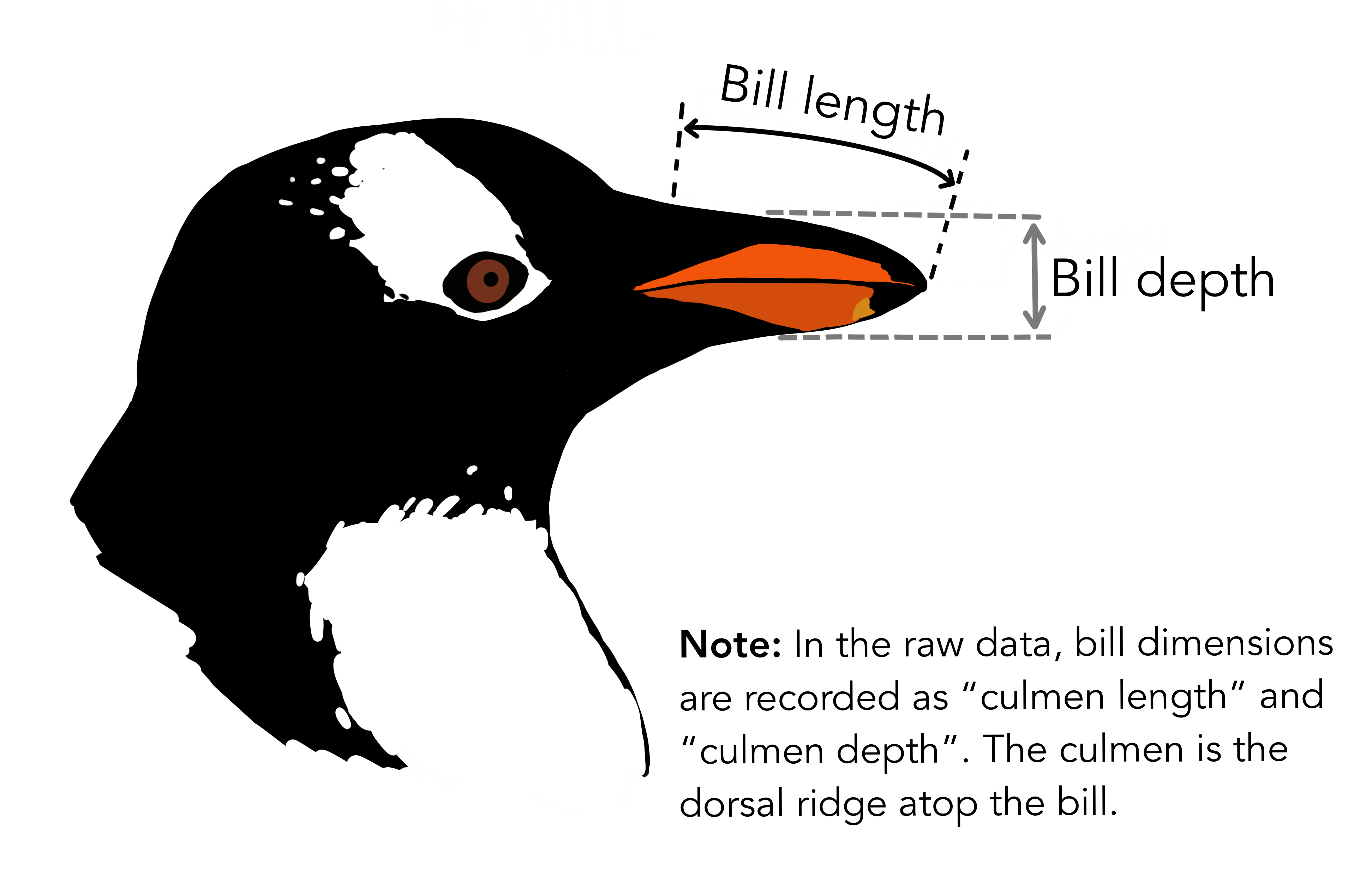2021-10-03

Summary

Data visualisation

• Grammar of graphics
• ggplot2

Next: Descriptive statistics

• pastecs::stat.desc
• dplyr::across

Meet the Palmer penguins

Original data collected and released by Dr. Kristen Gorman and the Palmer Station, Antarctica LTER, a member of the Long Term Ecological Research Network.

Horst AM, Hill AP, Gorman KB (2020). palmerpenguins: Palmer Archipelago (Antarctica) penguin data. R package version 0.1.0. doi:10.5281/zenodo.3960218.

library(palmerpenguins)Artwork by @allison_horst

Descriptive statistics

Quantitatively describe or summarize variables

• stat.desc from pastecs library
• base includes counts
• desc includes descriptive stats
• norm (default is FALSE) includes distribution stats
library(pastecs)

palmerpenguins::penguins %>%
dplyr::select(bill_length_mm, bill_depth_mm) %>%
pastecs::stat.desc() %>%
knitr::kable(digits = c(2, 2))

stat.desc output

bill_length_mm bill_depth_mm
nbr.val 342.00 342.00
nbr.null 0.00 0.00
nbr.na 2.00 2.00
min 32.10 13.10
max 59.60 21.50
range 27.50 8.40
sum 15021.30 5865.70
median 44.45 17.30
mean 43.92 17.15
SE.mean 0.30 0.11
CI.mean.0.95 0.58 0.21
var 29.81 3.90
std.dev 5.46 1.97
coef.var 0.12 0.12

stat.desc: basic

• nbr.val: overall number of values in the dataset
• nbr.null: number of NULL values – NULL is often returned by expressions and functions whose values are undefined
• nbr.na: number of NAs – missing value indicator
bill_length_mm bill_depth_mm
nbr.val 342.0 342.0
nbr.null 0.0 0.0
nbr.na 2.0 2.0
min 32.1 13.1
max 59.6 21.5
range 27.5 8.4
sum 15021.3 5865.7

stat.desc: basic

• min (also min()): minimum value in the dataset
• max (also max()): maximum value in the dataset
• range: difference between min and max (different from range())
• sum (also sum()): sum of the values in the dataset
bill_length_mm bill_depth_mm
nbr.val 342.0 342.0
nbr.null 0.0 0.0
nbr.na 2.0 2.0
min 32.1 13.1
max 59.6 21.5
range 27.5 8.4
sum 15021.3 5865.7

stat.desc: desc

• mean (also mean()): arithmetic mean, that is sum over the number of values not NA
• median (also median()): median, that is the value separating the higher half from the lower half the values
• mode()function is available: mode, the value that appears most often in the values
bill_length_mm bill_depth_mm
median 44.45 17.30
mean 43.92 17.15
SE.mean 0.30 0.11
CI.mean.0.95 0.58 0.21
var 29.81 3.90
std.dev 5.46 1.97
coef.var 0.12 0.12

Sample statistics

Assuming that the data in the dataset are a sample of a population

• SE.mean: standard error of the mean – estimation of the variability of the mean calculated on different samples of the data (see also central limit theorem)

• CI.mean.0.95: 95% confidence interval of the mean – indicates that there is a 95% probability that the actual mean is within that distance from the sample mean

Estimating variation

• var: variance ($$\sigma^2$$), it quantifies the amount of variation as the average of squared distances from the mean

$\sigma^2 = \frac{1}{n} \sum_{i=1}^n (\mu-x_i)^2$

• std.dev: standard deviation ($$\sigma$$), it quantifies the amount of variation as the square root of the variance

$\sigma = \sqrt{\frac{1}{n} \sum_{i=1}^n (\mu-x_i)^2}$

• coef.var: variation coefficient it quantifies the amount of variation as the standard deviation divided by the mean

dplyr::across

The dplyr verb across allows to apply summarise verbs on multiple columns. Instead of…

palmerpenguins::penguins %>%
# filter out raws with missing data
dplyr::filter(!is.na(bill_length_mm)) %>%
# summarise
dplyr::summarise(
avg_bill_len_mm = mean(bill_length_mm),
avg_bill_dpt_mm = mean(bill_depth_mm),
avg_flip_len_mm = mean(flipper_length_mm),
avg_body_mass_g = mean(body_mass_g)
) %>%
knitr::kable(digits = c(2, 2, 2, 2))
avg_bill_len_mm avg_bill_dpt_mm avg_flip_len_mm avg_body_mass_g
43.92 17.15 200.92 4201.75

dplyr::across

The verb across can also be used with mutate, to apply the same function to a number of columns

palmerpenguins::penguins %>%
# mutate cross columns
dplyr::mutate(
dplyr::across(
c(bill_length_mm, bill_depth_mm, flipper_length_mm),
# add 1 to all values in the columns above
function(x){ x / 25.4 }
)
) %>%
rename(
bill_length_in = bill_length_mm,
bill_depth_in = bill_depth_mm,
flipper_length_in = flipper_length_mm
)

dplyr::across

Old columns:

## # A tibble: 344 x 3
##   bill_length_mm bill_depth_mm flipper_length_mm
##            <dbl>         <dbl>             <int>
## 1           39.1          18.7               181
## 2           39.5          17.4               186
## # … with 342 more rows

New columns:

## # A tibble: 344 x 3
##   bill_length_in bill_depth_in flipper_length_in
##            <dbl>         <dbl>             <dbl>
## 1           1.54         0.736              7.13
## 2           1.56         0.685              7.32
## # … with 342 more rows

Summary

Descriptive statistics

• pastecs::stat.desc
• dplyr::across

Next: Exploring assumptions

• Normality
• Skewness and kurtosis
• Homogeneity of variance